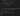# How to Convert List of Hexadecimal Values to Decimal

## Introduction#

Converting one type to another type is a common problem in scripting including converting list of hexadecimal values to decimal number. It will require more steps, thus it will be trickier.

In this blog post, we will walk you through how to convert list of Hex values to Decimal number.

## Solution#

This problem requires us to solve in two steps:

1. Iterating through the list
2. Converting Hex values to decimal

Since there are many ways to iterate and convert hex value to decimal, we will only give some examples. For more detail how to do each step, we suggest you to read article below.

### Converting from Array#

Array is one of data structures available in PowerShell. In this solution, we try to iterate from the array, then we convert each of hex value to decimal number.

``````
\$hexArray = "AD", "BE", "3C", "1F"

foreach (\$hex in \$hexArray) {
\$num = [Int32]"0x\$hex"

Write-Host \$num
}

``````

Below is the output:We can also iterate using `ForEach-Object` where it’s usually preceded by pipe `|`.

``````
\$hexArray = "AD", "BE", "3C", "1F"

\$hexArray | ForEach-Object {
\$num = [Int32]"0x\$_"

Write-Host \$num
}

``````

## Conclusion#

Converting list of hex values to decimal requires two steps. The first is iterating through the list and the second is converting hex value to decimal number.